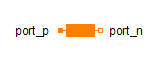Triggered Add - MapleSim Help

Add input to previous value of output at each triggerDescription The Triggered Add component adds the input u to the previous value of the output y when the trigger port has a rising edge. The output is initialized to ${y}_{0}$. If the reset port is enabled with use reset, then the output is reset to either set (if enabled via use set) or to ${y}_{0}$, whenever the reset port has a rising edge.Connections

 Name Description Modelica ID $u$ Integer input signal u $y$ Integer output signal y $\mathrm{trigger}$ Boolean input trigger $\mathrm{reset}$ Boolean input reset $\mathrm{set}$ Integer input setParameters

 Name Default Units Description Modelica ID use reset $\mathrm{false}$ True (checked) enables the reset port use_reset use set $\mathrm{false}$ True (checked) enables the set port use_set ${y}_{0}$ $0$ Initial and reset value of $y$ if set port is not used y_startModelica Standard Library The component described in this topic is from the Modelica Standard Library. To view the original documentation, which includes author and copyright information, click here.